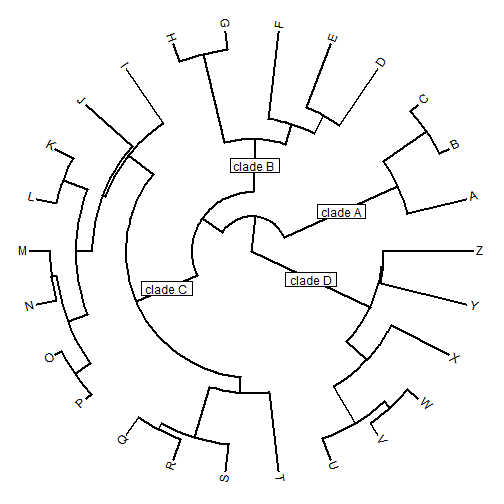## Friday, July 31, 2015

### Simulating an arbitrary shift in the diversification rate on a phylogeny

A R-sig-phylo reader recently asked if it is possible to simulate a shift in the rate of lineage diversification in some part of a tree (rather than simultaneously across all the lineages in a tree at a given time).

Now, I'll first say that I understand that methods to simulate various state-based models of diversification exist in the diversitree R package maintained by Rich Fitzjohn. If, however, one just wants to arbitrarily pick a point in the tree & switch to a different diversification rate (speciation and/or extinction rate) then this can also be done by merely simulating a complete tree under one process, going to the spot where you want the rate to change, pruning out the subtree tipward of that point, simulating a new subtree under your new desired process, and the pasting the two trees together.

Since this is easier said than done in R, the code below shows an example in which I first simulate a base tree with a birth rate of about `b~0.02`, a taxon-stop criterion of `20` and a time stop of `100`; then I (arbitrarily, just to demonstrate) pick a random lineage at 75% of the total tree height and call that my shift point; I prune that lineage out, and attach a new subtree in which I use a much higher birth-rate of about `b~0.12`.

Here's what that looks like:

``````library(phytools)
## set the birth rate based on n=20 & t=100
b1<-(log(20)-log(2))/100
b1
``````
``````##  0.02302585
``````
``````tree<-pbtree(b=b1,t=100,n=20)
``````
``````## simulating with both taxa-stop (n) and time-stop (t) is
## performed via rejection sampling & may be slow
##
##   4 trees rejected before finding a tree
``````
``````plotTree(tree,mar=c(3.1,0.1,0.1,0.1))
axis(1)
nodelabels()
``````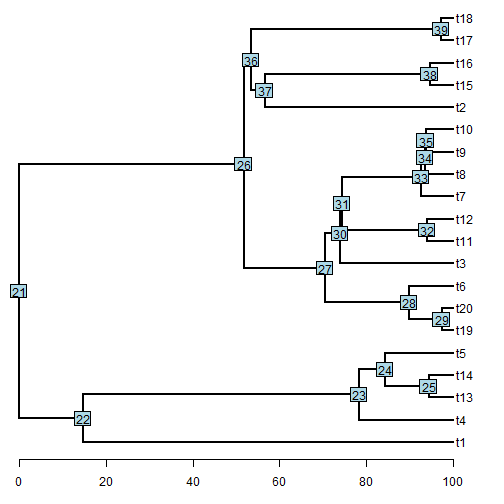``````## pick a split point at 75% of total tree height
pp<-0.75
H<-nodeHeights(tree)
node<-sample(tree\$edge[(H[,1]<pp*max(H))+
(H[,2]>pp*max(H))==2,2],1)
node
``````
``````##  28
``````
``````## split the tree & this point
tree<-splitTree(tree,split=list(node=node,
bp=pp*max(H)-H[which(tree\$edge[,2]==node),1]))[]
tree\$tip.label[tree\$tip.label=="NA"]<-""
plotTree(tree,mar=c(3.1,0.1,0.1,0.1))
axis(1)
nodelabels(node=tree\$tip.label=="",pch=19)
``````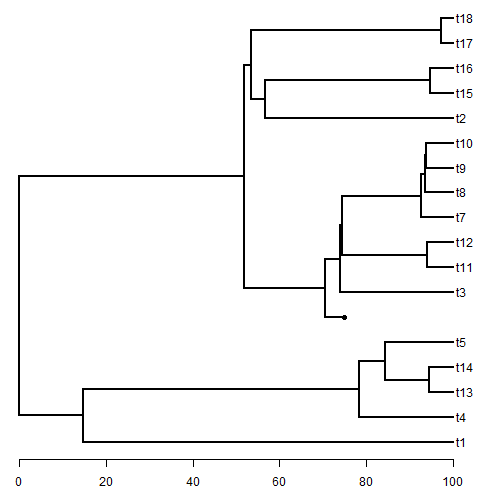``````## set the second desired birth-rate based on n=25 & t=25
## note this time we want to start with 1 lineage, not 2
b2<-log(25)/(max(H)-pp*max(H))
## simulate the "stem" of the new subtree
t1<-(max(H)-pp*max(H))
while(t1>=25) t1<-rexp(n=1,rate=b2)
ii<-which(tree\$edge[,2]==which(tree\$tip.label==""))
tree\$edge.length[ii]<-tree\$edge.length[ii]+t1
plotTree(tree,mar=c(3.1,0.1,0.1,0.1))
axis(1)
nodelabels(node=tree\$tip.label=="",pch=19)
``````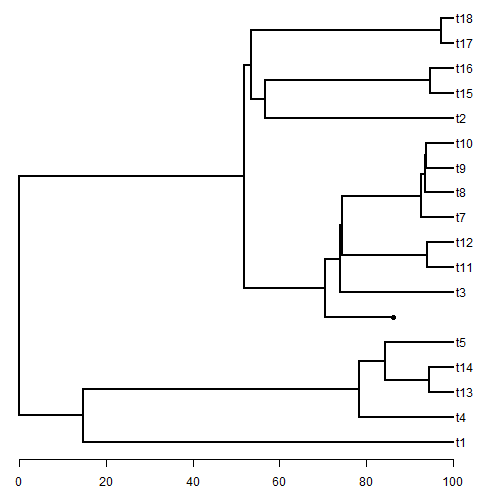``````## now simulate the rest of the derived subtree
t2<-pbtree(b=b2,t=max(nodeHeights(tree))-
nodeheight(tree,which(tree\$tip.label=="")),n=25)
``````
``````## simulating with both taxa-stop (n) and time-stop (t) is
## performed via rejection sampling & may be slow
##
##   103 trees rejected before finding a tree
``````
``````t2\$tip.label<-paste("s",1:Ntip(t2),sep="")
tree<-bind.tree(tree,t2,where=which(tree\$tip.label==""))
plotTree(tree,fsize=0.8,mar=c(3.1,0.1,0.1,0.1))
axis(1)
``````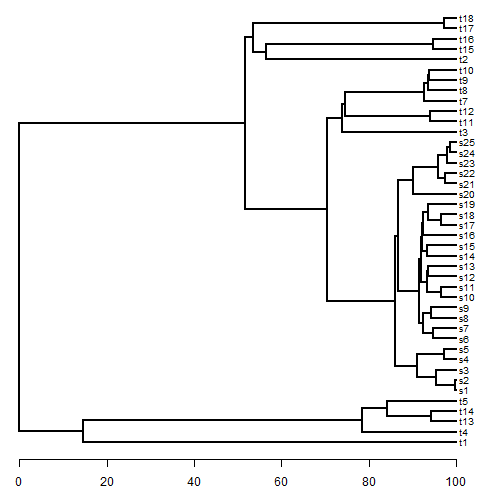``````## visualize the shift in diversification rate
ltt(tree,show.tree=TRUE)
``````
``````## Object of class "ltt" containing:
##
## (1) A phylogenetic tree with 42 tips and 41 internal nodes.
##
## (2) Vectors containing the number of lineages (ltt) and branching times (times) on the tree.
##
## (3) A value for Pybus & Harvey's "gamma" statistic of 2.8428, p-value = 0.0045.
``````
``````obj<-get("last_plot.phylo",envir=.PlotPhyloEnv)
points(x=pp*max(H),y=obj\$yy[findMRCA(tree,t2\$tip.label)],pch=19)
lines(rep(pp*max(H),2),par()\$usr[3:4],lty="dashed")
``````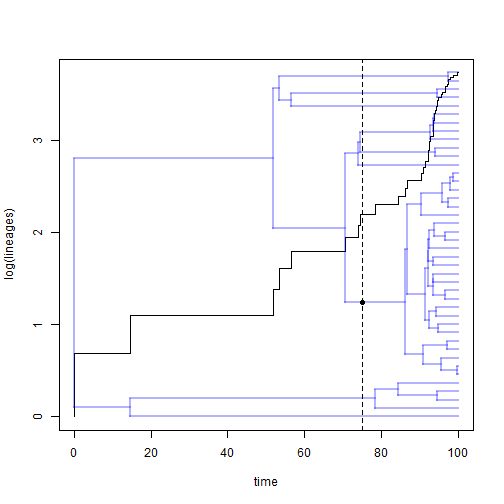That's it.

Obviously, this would have to be customized to whatever scenario the user is envisioning.

## Thursday, July 30, 2015

### Edge labels on a radial or fan tree

Today on R-sig-phylo a reader reported a bug in the way that edge labels are plotted using the ape function `edgelabels`.

This error is very easy to reproduce:

``````set.seed(8) ## for repeatability
library(phytools)
tree<-pbtree(n=26,tip.label=LETTERS)
plotTree(tree,type="fan")
``````
``````## setEnv=TRUE for this type is experimental. please be patient with bugs
``````
``````edgelabels()
``````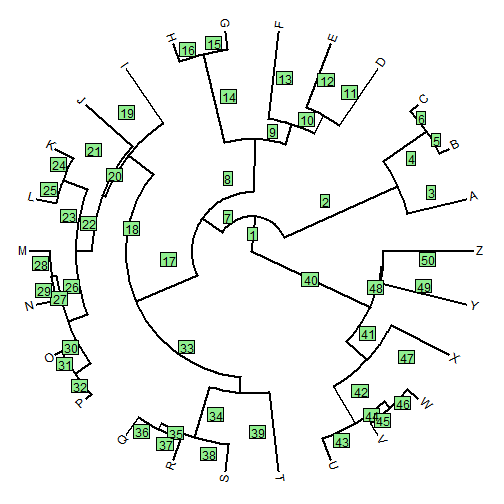The problem is fairly clear to see. Instead of plotting the edge labels mid-way along each edge, the function is plotting them midway between the daughter node of the edge - and the daughter node of the parent edge. This does not work (except for edges descended directly from the root node).

[Another peculiarity of the function is that the plotted numbers are, by default, the rows of `phy\$edge` - not, for instance, the index of the daughter node of each edge. Let's ignore that for now.]

Luckily, Klaus Schliep, a postdoc presently working in my lab & author of the phangorn package quickly leapt to the rescue with a complete bug fix. However, a little too late I also had written a little code which, though not as complete as Klaus's fix, nonetheless might be instructive (with comments below) on how these functions work.

Here is my function, which by default plots numbers corresponding to the daughter of each edge - not the row of `phy\$edge`:

``````edgelabels.fan<-function(text=NULL,edge=NULL,cex=1){
## get the last plotted "phylo" object - created by
## plot.phylo, plotTree, and other methods
obj<-get("last_plot.phylo",envir=.PlotPhyloEnv)
## these are the positions of the nodes & tips in the plotted
## tree
nx<-obj\$xx[obj\$edge[,2]]
ny<-obj\$yy[obj\$edge[,2]]
## this function computes the length of each edge from the
## object we pulled, above
foo<-function(e,x,y) sqrt(x[e]^2+y[e]^2)-
sqrt(x[e]^2+y[e]^2)
l<-apply(obj\$edge,1,foo,x=obj\$xx,y=obj\$yy)
## now let's get the x & y coordinates of the midpoint
## of each edge
x<-nx-sign(nx)*0.5*l*cos(atan(ny/nx))
y<-ny-sign(nx)*0.5*l*sin(atan(ny/nx))
## some bookkeeping
ii<-order(as.numeric(names(x)))
x<-x[ii]
y<-y[ii]
if(is.null(edge)) edge<-c(1:obj\$Ntip,2:obj\$Nnode+obj\$Ntip)
x<-x[as.character(edge)]
y<-y[as.character(edge)]
if(is.null(text)) text<-names(x)
if(length(text)!=length(edge)){
cat("Warning: edge & text should be the same length.\n\n")
text<-as.character(edge)
}
## let's plot our edge labels
rectangles=cbind(1.2*cex*strwidth(text),
1.4*cex*strheight(text)))
text(text,x=x,y=y,cex=cex)
}
``````

OK, now we might as well try it out:

``````plotTree(tree,type="fan")
``````
``````## setEnv=TRUE for this type is experimental. please be patient with bugs
``````
``````edgelabels.fan()
``````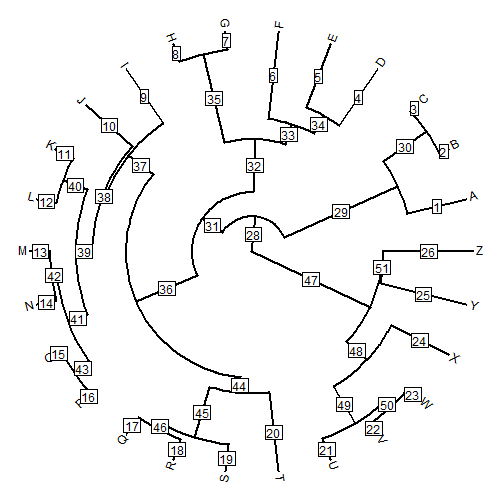or, alternatively:

``````plotTree(tree,type="fan")
``````
``````## setEnv=TRUE for this type is experimental. please be patient with bugs
``````
``````edgelabels.fan(edge=c(29,32,36,47),